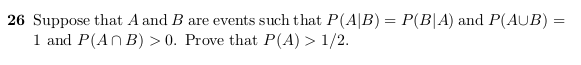• Using the formula for conditional probability for events A and B:

$$P(A | B) = P(A \cap B) / P(B)$$ and $$P(B | A) = P(B \cap A) / P(A)$$

we can rewrite the equality in the question to be:

1. $$P(A \cap B)P(A) = P(B \cap A)P(B)$$ using cross-multiplication.
• Per the probability communitive law for two events, $$P(A \cap B) = P(B \cap A)$$

thus we can divide the $$P(A \cap B)$$ on both sides to get

1. $$P(A) = P(B)$$
• We’re not done yet. We have to show $$P(A) > 1/2$$ and to do this, recall the formula

of $$P(A \cup B)$$ that is the probability of either of the two events A and B happening

is:

• $$P(A \cup B) = P(A) + P(B) - P(A \cap B)$$

• We can solve for P(B) to get

• $$P(A \cup B) + P(A \cap B) - P(A) = P(B)$$ and plug it into b. to get

• $$P(A) = P(A \cup B) + P(A \cap B) - P(A)$$

• Adding $$P(A)$$ on both sides gives

• $$2P(A) = P(A \cup B) + P(A \cap B)$$

• Dividing on both sides by 2 gives

• $$P(A) = P(A \cup B)/2 + P(A \cap B)/2$$

• $$P(A \cup B) = 1$$ as per the information given so

• $$P(A) = 1/2 + P(A \cap B)/2$$

• Finally note that the second operand $$P(A \cap B)/2$$ will always be within (0,1]

• so $$P(A) \gt 1/2$$